John Cassel

## Probabilistic Programming with Stochastic MemoizationNBCDFPDF

### Implementing Nonparametric Bayesian Inference

Probabilistic programming is a programming language paradigm receiving both government support  and the attention of the popular technology press . Probabilistic programming concerns writing programs with segments that can be interpreted as parameter and conditional distributions, yielding statistical findings through nonstandard execution. Mathematica not only has great support for statistics, but has another language feature particular to probabilistic language elements, namely memoization, which is the ability for functions to retain their value for particular function calls across parameters, creating random trials that retain their value. Recent research has found that reasoning about processes instead of given parameters has allowed Bayesian inference to undertake more flexible models that require computational support. This article explains this nonparametric Bayesian inference, shows how Mathematica‘s capacity for memoization supports probabilistic programming features, and demonstrates this capability through two examples, learning systems of relations and learning arithmetic functions based on output.

### Nonparametric Bayesian Inference

Bayesian statistics are an orderly way of finding the likelihood of a model from data, using the likelihood of the data given the model. From spam detection to medical diagnosis, spelling correction to forecasting economic and demographic trends, Bayesian statistics have found many applications, and even praise as mental heuristics to avoid overconfidence. However, at first glance Bayesian statistics suffer from an apparent limit: they can only make inferences about known factors, bounded to conditions seen within the data, and have nothing to say about the likelihood of new phenomena . In short, Bayesian statistics are apparently withheld to inferences about the parameters of the model they are provided.

Instead of taking priors over factors of the model itself, we can say that we are taking priors over factors in the process involving how the data was generated. These stochastic process priors give the modeler a way to talk about factors that have not been directly observed. These nonobservable factors include the likely rate at which further factors might be seen, given further observation and underlying categories or structures that might generate the data being observed. For example, in statistics problems we are often presented with drawing marbles of different colors from a bag, and given randomly drawn samples, we might talk about the most likely composition of the bag and the range of likely compositions. However, suppose we had a number of bags, and we drew two marbles each from three of them, discovering two red marbles, two green marbles, and two yellow marbles . If we were to draw marbles from yet another bag, we might expect two marbles identical in color, of a color we have not previously observed. We do not know what this color is, and in this sense we have made a nonparametric inference about the process that arranged the marbles between bags.

The ability to talk about nonobserved parameters is a leap in expressiveness, as instead of explicitly specifying a model for all parameters, a model utilizing infinite processes expands to fit the given data. This should be regarded similarly to the advantages afforded by linked data structures in representing ordinary data. A linked list has a potentially infinite capacity; its advantage is not that we have an infinite memory, but an abstract flexibility to not worry too much about maintaining its size appropriately. Similarly, an infinite prior models the growth we expect to discover .

Here are two specific processes that are useful for a number of different problems. These two processes are good for modeling unknown discrete categories and sets of features, respectively. In both of these processes, suppose that we can take samples so that there are no dependencies in the order that we took them, or in other words that the samples are exchangeable. Both of these processes also make use of a concentration parameter,. As we look at more samples, we expect the number of new elements we discover to diminish, but not disappear, as our observations establish a lower frequency of occurrence for unobserved elements. The concentration parameter establishes the degree to which the proportions are concentrated, with lowindicating a distribution concentrated on a few elements, and highindicating a more dispersed concentration.

First, let us look into learning an underlying system of categories. In a fixed set of categories of particular likelihood, the probability of a given sample in a particular category corresponds to the multinomial distribution, the multiparameter extension of the Bernoulli distribution. The conjugate prior, or the distribution that gives a Bayesian estimate of which multinomial distribution produced a given sample, is the Dirichlet distribution, itself the multivariable extension of the beta distribution. To create an infinite Dirichlet distribution, or rather a Dirichlet process, one can simply have a recursive form of the beta where the likelihood of a given category is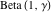. To use a Dirichlet process as a prior, it is easier to manipulate in the form of a Chinese restaurant process (CRP) . Suppose we want to know the likelihood that the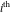sample is a member of category. If the category is new, then that probability corresponds to the size of the concentration parameter in ratio to the count of the samples taken: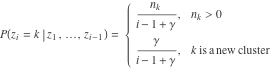The implementation of this function is straightforward. The use of a parameterized random number function allows for the use of the algorithm in common random number comparison between simulation scenarios , as well as for estimation through Markov chain Monte Carlo, about which more will be said later.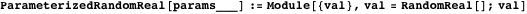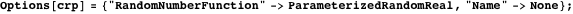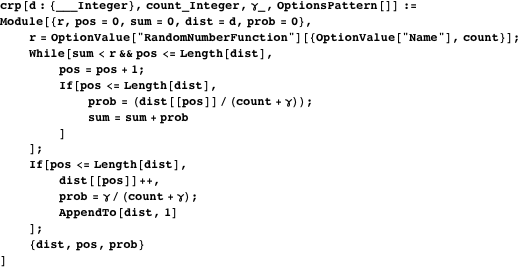In the second process, suppose we are interested in the sets of features observed in sets of examples. For example, suppose we go to an Indian food buffet and are unfamiliar with the dishes, so we observe the selected items that our fellow patrons have chosen. Supposing one overall taste preference, we might say that the likelihood of a dish’s being worth selecting is proportional to the number of times it was observed, but if there are not many examples we should also try some additional dishes that were not tried previously. This process, called the Indian buffet process , turns out to be equivalent to a beta process prior . Suppose we want to know the likelihood of whether a given featureis going to be found in the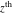sample. Then, the likelihoods can be calculated directly from other well-understood distributions: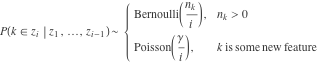Both of these processes are suitable as components in mixture models. Suppose we are conducting a phone poll of a city and ask the citizens we talk to about their concerns. Each person will report their various civic travails. We expect for each person to have their own varying issues, but also for there to be particular groups of concern for different neighborhoods and professional groups. In other words, we expect to see an unknown set of features emerge from an unknown set of categories. Then, we might use a CRPIBP mixture distribution to help learn those categories from the discovered feature sets.

Nonparametric inference tasks are particularly suited for computational support. What we would like to do is describe a space of potential mixture models that may describe the underlying data-generation processes and allow the inference of their likelihood without explicitly generating the potential structures of that space. Probabilistic programming is the use of language-specific support to aid in the process of statistical inference. This article shows that Mathematica has features that readily enable the sort of probabilistic programming that supports nonparametric inference.

### Probabilistic Programming

Probabilistic programming is the use of language-specific support to aid in the process of statistical inference. Unlike statistical libraries, the structure of the programming language itself is used in the inference process . Although Mathematica increasingly has the kinds of structures that support probabilistic programming, we are not going to focus on those features here. Instead, we will see how Mathematica‘s natural capacity for memoization allows it to be very easily extended to write probabilistic programs that use stochastic memoization as a key abstraction. In particular, we are going to look at Church, a Lisp-variant with probabilistic query and stochastic memoization constructs . Let us now explain stochastic memoization and then look at how to implement Metropolis-Hastings querying, which uses memoization to help implement Markov chain Monte Carlo-driven inference.

#### Stochastic Memoization

Stochastic memoization simply means remembering probabilistic events that have already occurred. Suppose we say that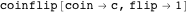is the first flip of coin c. In the first call, it may return Heads or Tails, depending on a likelihood imposed to coin c, but in either case it is constrained in later calls to return the same value. Once undertaken, the value of a particular random event is determined.

In Church, this memoization is undertaken explicitly through its mem operator. Church’s flip function designates a Bernoulli trial with the given odds, with return values 0 and 1. Here is an example of a memoized fair coin flip in Church.

`(define coinflip (mem (lambda (coin flip) (flip 0.5))))`

Mathematica allows for a similar memoization by incorporating a Set within a SetDelayed.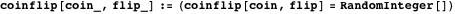Let us now look to a more complicated case. Earlier, we discussed the Dirichlet process. Church supports a DPmem operator for creating functions that when given a new example either returns a previously obtained sample according to the CRP or takes a new sample, depending upon the category assignment, and returns the previously seen argument. Here is a similar function in Mathematica, called GenerateMemCRP. Given a random function, we first create a memoized version of that function based on the category index of the CRP. Then, we create an empty initial CRP result, for which a new sample is created and memoized every time a new input is provided, potentially also resampling the provided function if a prediscovered category is provided.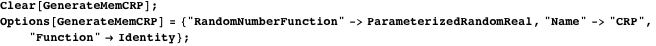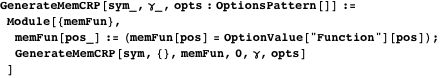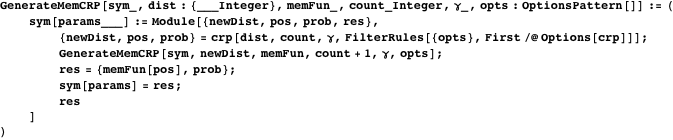For example, let us now take a sampling from categories that have a parameter distributed according to the standard normal distribution. Here we see outputs in a typical range for a standard normal, but with counts favoring resampling particular results according to the sampled frequency of the corresponding category.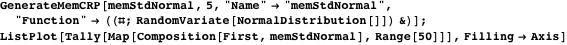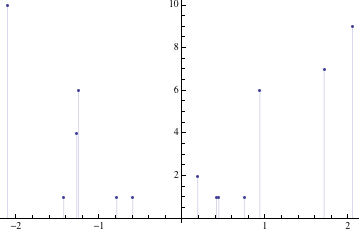Memoization implies that if we provide the same inputs, we get the same results.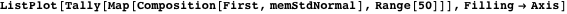#### Metropolis-Hastings Querying

Inference is the central operation of probabilistic programming. Conditional inference is implemented in Church through its various query operations. These queries uniformly take four sets of arguments: query algorithm-specific parameters, a description of the inference problem, a condition to be satisfied, and the expression we want to know the distribution of given that condition. Let us motivate the need for a Mathematica equivalent to the Church query operator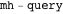by explaining other queries that are trivial to implement in Mathematica but that are not up to certain inference tasks.

Direct calculation is the most straightforward approach to conditional inference. However, sometimes we cannot directly compute the conditional likelihood, but instead have to sample the space. The easiest way to do so is rejection sampling, in which we generate a random sample for all random parameters to see if it meets the condition to be satisfied. If it does, its value is worth keeping as a sample of the distribution, and if it does not, we discard it entirely, proceeding until we are satisfied that we have found the distribution we intend.

There is a problem with rejection sampling, namely that much of the potential model space might be highly unlikely and that we are throwing away most of the samples. Instead of doing that, we can start at a random place but then, at each step, use that sample to find a good sample for the underlying distribution . So, for a sample, we are interested in constructing a transition operator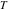yielding a new sample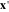, and constructing that operator such that for the underlying distribution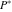, the transition operator is invariant with respect to distribution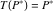, or in other words, that the transition operator forms a Markov chain. For our transition operator, we first choose to generate a random proposal,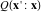, where a simple choice is the normally distributed variation along all parameters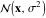, and then accept that proposal with likelihood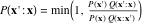, so that we are incorporating less-likely samples at exactly the rate the underlying distribution would provide. After some initial samples of random value, we will have found the region for which the invariance property holds. Due to the use of applying random numbers to a Markov chain, this algorithm is called Markov chain Monte Carlo, or MCMC.

The following procedure is intended to be the simplest possible implementation of MCMC using memoization (for further considerations see [13, 14]). There is a trade-off in the selection of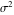, such that if it is too large, we rarely accept anything and would effectively be undertaking rejection sampling, but if it is too small, we tend to stay in a very local area of the algorithm. One way to manage this trade-off is to controlby aiming for a given rejection rate, which is undertaken here.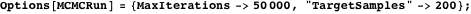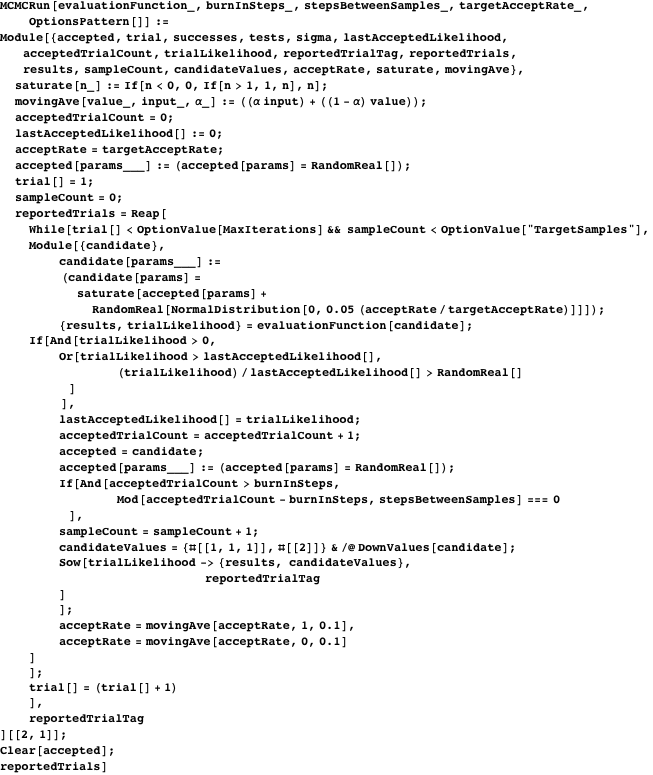We now see why we constructed the CRP functions to accept random number functions: it lets us create evaluation functions suitable for MCMC.

### Examples

Let us look to see how we might apply these examples. First, we are going to look at the infinite relational model, which demonstrates how to use the CRP to learn underlying categories from relations. Then, we will look at learning arithmetic expressions based upon particular inputs and outputs, which demonstrates using probabilistic programming in a recursive setting.

#### The Infinite Relational Model

Suppose we are given some set of relations in the form of predicates, and we want to infer category memberships based on those relations. The infinite relational model (IRM) can construct infinite category models for processing arbitrary systems of relational data . Suppose now we have some specific instances of objects,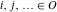, and a few specific statements about whether a given-ary relation,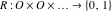, holds between them or not. Given a prior of how concentrated categories are,, and a prior for the sharpness of a relation to holding between members of various types,, we would like to learn categories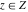and relational likelihoods, such that we can infer category memberships for objects and the likelihood of unobserved relationships holding between them, which corresponds to the following model structure.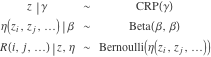Below we provide a sampler to calculate this, which first sets up memoization for category assignment, next memoization for relational likelihood, and then a function for first evaluating the object to category sampling and then the predicate/category sampling. Then, the function merely calculates the likelihood sampling along the way, returning the object to category memberships and the likelihood.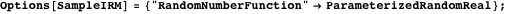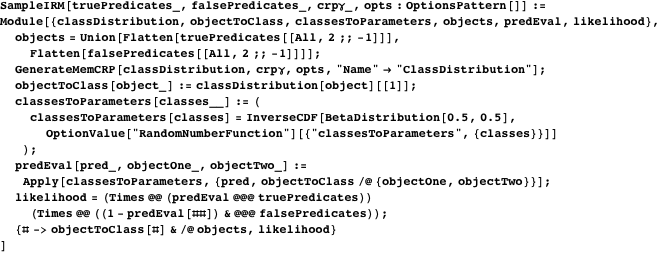To understand how this works, let us look at an example. Suppose that there are two elementary schools, one for girls and one for boys, and that they both join together for high school. However, there is a new teacher who does not know this about the composition of incoming classes. This teacher finds another teacher and asks if they know who knows whom. This more experienced teacher says yes, but not why, and the younger teacher asks a series of questions about who knows whom, to the confusion of the older teacher, who does not understand why the younger teacher does not know (we have all had conversations like this). One potential set of such questions might yield the following answers. Notice that there is a deficiency in these questions; namely, the new teacher never asks if a boy knows a girl.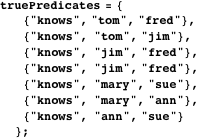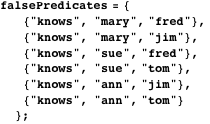Given these samples, let us now perform a Metropolis-Hastings query to see if we can recover these categories. The result of a particular sample is a list of rules, where the left side of each rule is the likelihood of the given predicates to hold given the sampled model, and the right side is the sampled model in a two-element list. In this two-element list characterizing the sampled model, the first element is the result as provided by the evaluation method, and the second element contains the random values parameterizing the model.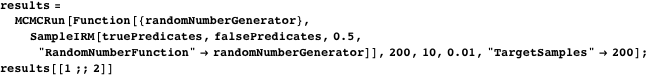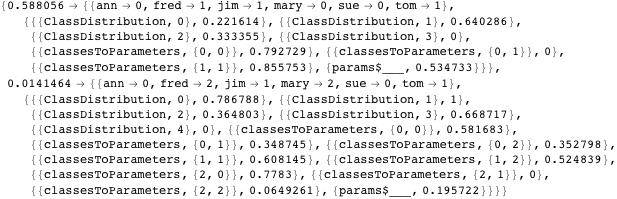Given these samples, let us now find a list of categories that fit them. Normalize the weight of each example by its likelihood, filter out the sampling information, and gather the common results together.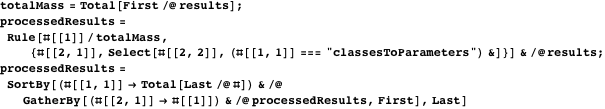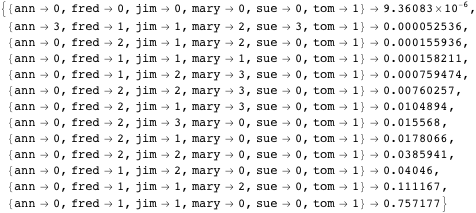Removing the specific category assignments and determining for each person whether they are in the same category as each other, we see that we have a complete and accurate estimate for who knows whom.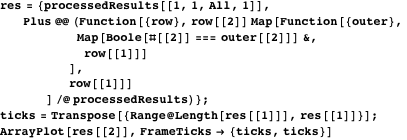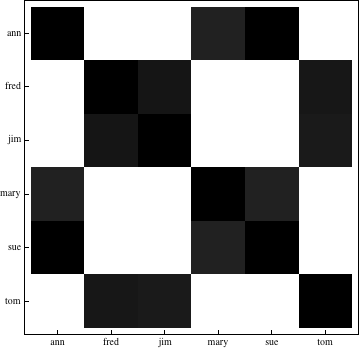#### Learning Simple Arithmetic Expressions

There is no more idiomatic example of probabilistic programming than probabilistically generated programs. Here, we show how to implement learning simple arithmetic expressions. A Church program for undertaking this is as follows . First, it defines a function for equality that is slightly noisy, creating a gradient that is easier to learn than strict satisfaction. Next, it creates a random arithmetic expression of nested addition and subtraction with a single variable and integers from 0 to 10 as terminals. Then, it provides a utility for evaluating symbolically constructed expressions. Finally, it demonstrates sampling a program with two results that are consistent with adding 2 to the input.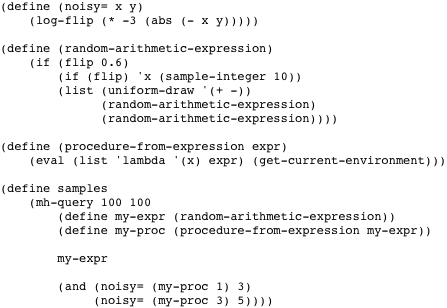Let us now construct an equivalent Mathematica program. First, we will construct an equivalent noisy equality operator.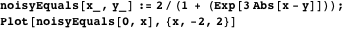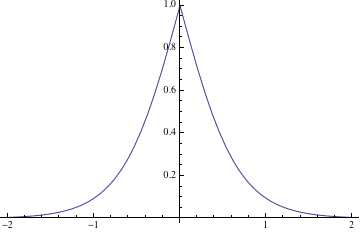Next, here is an equivalent program for generating random programs. By recursively indexing each potential branch of the program, we can assure that common random number and MCMC algorithms will correctly assign a random number corresponding to that exact part of the potential program. We also explicitly limit the size of the tree.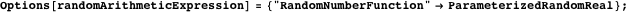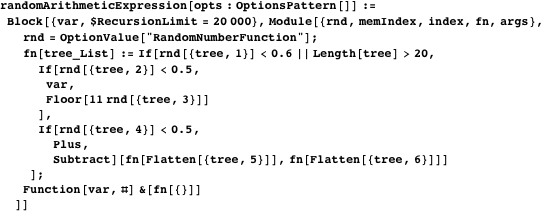Now we make a Metropolis-Hastings query and process the results down to the found expression and its calculated likelihood.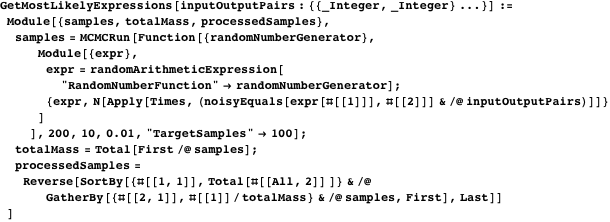Given only one example, we cannot tell very much, but are pleased that the simplest, yet correct, function is the one rated most likely. Interestingly, the first six expressions are valid despite the noisy success condition.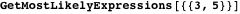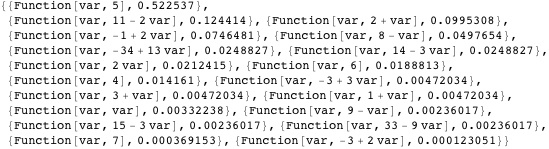With two inputs, the only viable expression is the one found.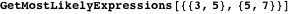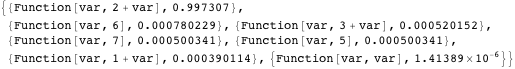### Summary

We have now seen how to implement nonparametric Bayesian inference with Mathematica‘s memoization features. Nonparametric Bayesian inference extends Bayesian inference to processes, allowing for the consideration of factors that are not directly observable, creating flexible mixture models with similar advantages to flexible data structures. We see that Mathematica‘s capacity for memoization allows for the implementation of nonparametric sample generation and for Markov chain sampling. This capacity was then demonstrated with two examples, one for discovering the categories underlying particular observed relations and the other for generating functions that matched given results.

### Conclusion

Probabilistic programming is a great way to undertake nonparametric Bayesian inference, but one should not confuse language-specific constructs with the language features that allow one to undertake it profitably. Through Mathematica‘s memoization capabilities, it is readily possible to make inferences over flexible probabilistic models.

### References

  DARPA. “Probabilistic Programming for Advancing Machine Learning (PPAML).” Solicitation Number: DARPA-BAA-13-31. (Aug 8, 2013) www.fbo.gov/utils/view?id=a7bdf07d124ac2b1dda079de6de2eb78.  B. Cronin. “What Is Probabilistic Programming?” O’Reilly Radar (blog). (Aug 8, 2013) radar.oreilly.com/2013/04/probabilistic-programming.html.  D. Fidler, “Foresight Defined as a Component of Strategic Management,” Futures, 43(5), 2011 pp. 540-544. doi:10.1016/j.futures.2011.02.005.  C. Kemp, A. Perfors, and J. B. Tenenbaum, “Learning Overhypotheses with Hierarchical Bayesian Models,” Developmental Science, 10(3), 2007 pp. 307-321. doi:10.1111/j.1467-7687.2007.00585.x.  M. I. Jordan, “Bayesian Nonparametric Learning: Expressive Priors for Intelligent Systems,” in Heuristics, Probability, and Causality: A Tribute to Judea Pearl, (R. Dechter, H. Geffner, and J. Y. Halpern, eds.) London: College Publications, 2010.  J. Pitman, Combinatorial Stochastic Processes (Lecture Notes in Mathematics 1875), Berlin: Springer-Verlag, 2006.  A. Law and D. Kelton, Simulation Modeling and Analysis, 3rd ed., Boston: McGraw-Hill, 2000.  T. Griffiths and Z. Ghahramani, “Infinite Latent Feature Models and the Indian Buffet Process,” in Proceedings of the Eighteenth Annual Conference on Neural Information Processing Systems (NIPS 18), Whistler, Canada, 2004. books.nips.cc/papers/files/nips18/NIPS2005_0130.pdf.  R. Thibaux and M. I. Jordan, “Hierarchical Beta Processes and the Indian Buffet Process,” in Proceedings of the Eleventh International Conference on Artificial Intelligence and Statistics (AISTATS 2007), San Juan, Puerto Rico, 2007. jmlr.org/proceedings/papers/v2/thibaux07a/thibaux07a.pdf.  N. D. Goodman. “The Principles and Practice of Probabilistic Programming,” in Proceedings of the 40th Annual ACM SIGPLAN-SIGACT Symposium on Principles of Programming Languages, (POPL 2013) Rome, Italy, 2013 pp. 399-402. doi:10.1145/2429069.2429117.  N. D. Goodman, V. K. Mansinghka, D. Roy, K. Bonawitz, and J. B. Tenenbaum, “Church: A Language for Generative Models,” in Proceedings of the Twenty-Fourth Conference on Uncertainty in Artificial Intelligence (UAI2008), Helsinki, Finland, 2008. www.auai.org/uai2008/UAI_camera_ready/goodman.pdf.  I. Murray. Markov Chain Monte Carlo . (Aug 8, 2013) videolectures.net/mlss09uk_murray_mcmc.  D. J. C. MacKay, Information Theory, Inference, and Learning Algorithms, Cambridge, UK: Cambridge University Press, 2003. www.inference.phy.cam.ac.uk/itila/book.html.  C. Robert and G. Casella, Monte Carlo Statistical Methods, 2nd ed, New York: Springer, 2004.  C. Kemp, J. B. Tenenbaum, T. L. Griffiths, T. Yamada, and N. Ueda, “Learning Systems of Concepts with an Infinite Relational Model,” in Proceedings of the 21st National Conference on Artificial Intelligence (AAAI-06), Boston, MA, 2006. www.aaai.org/Papers/AAAI/2006/AAAI06-061.pdf.  N. D. Goodman, J. B. Tenenbaum, T. J. O’Donnell, and the Church Working Group. “Probabilistic Models of Cognition.” (Aug 8, 2013) projects.csail.mit.edu/church/wiki/Probabilistic_Models_of _Cognition. J. Cassel, “Probabilistic Programming with Stochastic Memoization,” The Mathematica Journal, 2014. dx.doi.org/doi:10.3888/tmj.16-1.